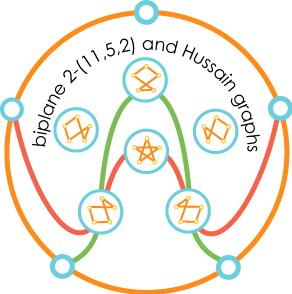# BiplanesThis is a generator-only picture of the biplane with parameters 2-(11,5,2). This geometry has 11 points and 11 lines with 5 points on every line and 5 lines passing through every point. Furthermore, 2 distinct points are contained in exactly two distinct lines and 2 distinct lines intersect in exactly two distinct points. The 11 blue labels correspond to the 11 points of the biplane. The lines are "generated" by the three distinguished lines (orange, red and green) by rotating the diagram around its center 72 degrees at a time.

Hussain graphs

 Associated with every biplane is a set of graphs, the so-called Hussain graphs, that encode all the information about the biplane. In the case of our biplane there are 6 Hussain graphs on a common set of five yellow points. The points in the middle of the diagram are labelled with these graphs. Note that any two of the graphs have exactly two edges in common. What is the connection between the biplane and its associated Hussain graphs?